# MagnetostaticResults

Magnetostatic solution and derived quantities

Since R2021a

## Description

A `MagnetostaticResults` object contains the magnetic potential, magnetic field, magnetic flux density, and mesh values in a form convenient for plotting and postprocessing.

The magnetic potential, magnetic field, and magnetic flux density are calculated at the nodes of the triangular or tetrahedral mesh generated by `generateMesh`. Magnetic potential values at the nodes appear in the `MagneticPotential` property. Magnetic field values at the nodes appear in the `MagneticField` property. Magnetic flux density values at the nodes appear in the `MagneticFluxDensity` property.

To interpolate the magnetic potential, magnetic field, and magnetic flux density to a custom grid, such as the one specified by `meshgrid`, use the `interpolateMagneticPotential`, `interpolateMagneticField`, and `interpolateMagneticFlux` functions.

## Creation

Solve a magnetostatic problem using the `solve` function. This function returns a solution as a `MagnetostaticResults` object.

## Properties

expand all

Magnetic potential values at nodes, returned as a vector for a 2-D problem or an `FEStruct` object for a 3-D problem. The properties of this object contain the components of the magnetic potential at nodes.

Magnetic field values at nodes, returned as an `FEStruct` object. The properties of this object contain the components of the magnetic field at nodes.

Magnetic flux density values at nodes, returned as an `FEStruct` object. The properties of this object contain the components of the magnetic flux density at nodes.

Finite element mesh, returned as an `FEMesh` object. For details, see FEMesh Properties. For a 3-D model, the mesh must be linear.

## Object Functions

 `interpolateMagneticPotential` Interpolate magnetic potential in magnetostatic result at arbitrary spatial locations `interpolateMagneticField` Interpolate magnetic field in magnetostatic result at arbitrary spatial locations `interpolateMagneticFlux` Interpolate magnetic flux density in magnetostatic result at arbitrary spatial locations

## Examples

collapse all

Solve a 2-D electromagnetic problem on a geometry representing a plate with a hole in its center. Plot the resulting magnetic potential and field distribution.

Create an electromagnetic model for magnetostatic analysis.

`emagmodel = createpde("electromagnetic","magnetostatic");`

Import and plot the geometry representing a plate with a hole.

```importGeometry(emagmodel,"PlateHolePlanar.stl"); pdegplot(emagmodel,"EdgeLabels","on")```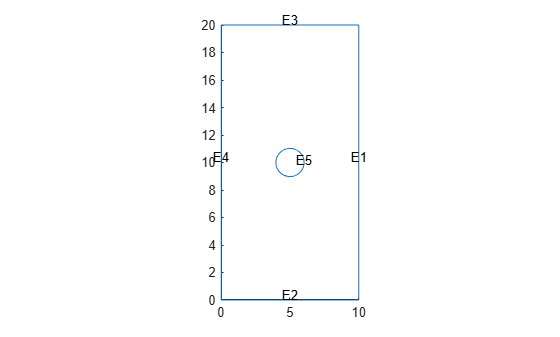Specify the vacuum permeability value in the SI system of units.

`emagmodel.VacuumPermeability = 1.2566370614e-6;`

Specify the relative permeability of the material.

`electromagneticProperties(emagmodel,"RelativePermeability",5000);`

Apply the magnetic potential boundary conditions on the edges framing the rectangle and the circle.

```electromagneticBC(emagmodel,"MagneticPotential",0,"Edge",1:4); electromagneticBC(emagmodel,"MagneticPotential",0.01,"Edge",5);```

Specify the current density for the entire geometry.

`electromagneticSource(emagmodel,"CurrentDensity",0.5);`

Generate the mesh.

`generateMesh(emagmodel);`

Solve the model.

`R = solve(emagmodel)`
```R = MagnetostaticResults with properties: MagneticPotential: [1218x1 double] MagneticField: [1x1 FEStruct] MagneticFluxDensity: [1x1 FEStruct] Mesh: [1x1 FEMesh] ```

Plot the magnetic potential and field.

```pdeplot(emagmodel,"XYData",R.MagneticPotential, ... "FlowData",[R.MagneticField.Hx ... R.MagneticField.Hy]) axis equal```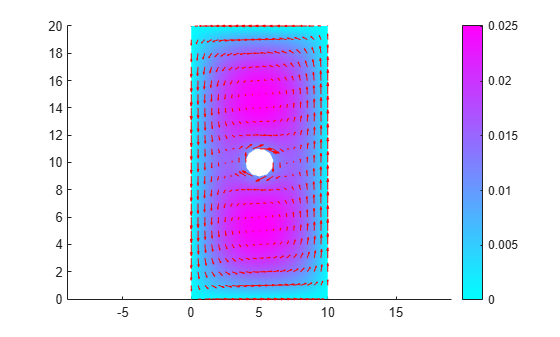Solve a 3-D electromagnetic problem on a geometry representing a plate with a hole in its center. Plot the resulting magnetic potential and field distribution.

Create an electromagnetic model for magnetostatic analysis.

`emagmodel = createpde("electromagnetic","magnetostatic");`

Import and plot the geometry representing a plate with a hole.

```importGeometry(emagmodel,"PlateHoleSolid.stl"); pdegplot(emagmodel,"FaceLabels","on","FaceAlpha",0.3)```Specify the vacuum permeability value in the SI system of units.

`emagmodel.VacuumPermeability = 1.2566370614e-6;`

Specify the relative permeability of the material.

`electromagneticProperties(emagmodel,"RelativePermeability",5000);`

Apply the magnetic potential boundary conditions on the side faces and the face bordering the hole.

```electromagneticBC(emagmodel,"MagneticPotential",[0;0;0],"Face",3:6); electromagneticBC(emagmodel,"MagneticPotential",[0;0;0.01],"Face",7);```

Specify the current density for the entire geometry.

`electromagneticSource(emagmodel,"CurrentDensity",[0;0;0.5]);`

Generate the mesh.

`generateMesh(emagmodel);`

Solve the model.

`R = solve(emagmodel)`
```R = MagnetostaticResults with properties: MagneticPotential: [1x1 FEStruct] MagneticField: [1x1 FEStruct] MagneticFluxDensity: [1x1 FEStruct] Mesh: [1x1 FEMesh] ```

Plot the z-component of the magnetic potential.

`pdeplot3D(emagmodel,"ColormapData",R.MagneticPotential.Az)`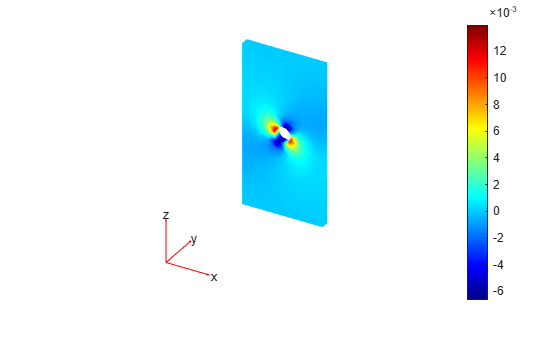Plot the magnetic field.

```pdeplot3D(emagmodel,"FlowData",[R.MagneticField.Hx ... R.MagneticField.Hy ... R.MagneticField.Hz])```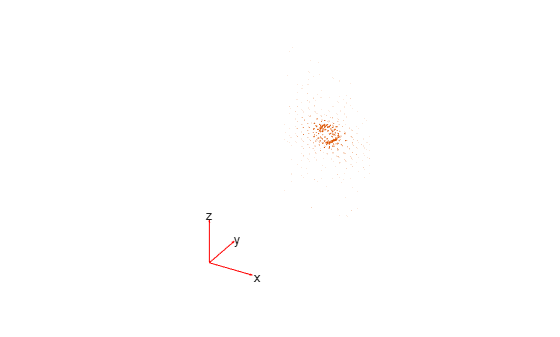Use a solution obtained by performing a DC conduction analysis to specify current density for a magnetostatic model.

Create an electromagnetic model for DC conduction analysis.

`emagmodel = createpde("electromagnetic","conduction");`

Import and plot a geometry representing a plate with a hole.

```gm = importGeometry(emagmodel,"PlateHoleSolid.stl"); pdegplot(gm,"FaceLabels","on","FaceAlpha",0.3)```Specify the conductivity of the material.

`electromagneticProperties(emagmodel,"Conductivity",6e4);`

Apply the voltage boundary conditions on the left, right, top, and bottom faces of the plate.

`electromagneticBC(emagmodel,"Voltage",0,"Face",3:6);`

Specify the surface current density on the face bordering the hole.

`electromagneticBC(emagmodel,"SurfaceCurrentDensity",100,"Face",7);`

Generate the mesh.

`generateMesh(emagmodel);`

Solve the model.

`R = solve(emagmodel);`

Change the analysis type of the model to magnetostatic.

`emagmodel.AnalysisType = "magnetostatic";`

This model already has a quadratic mesh that you generated for the DC conduction analysis. For a 3-D magnetostatic model, the mesh must be linear. Generate a new linear mesh. The `generateMesh` function creates a linear mesh by default if the model is 3-D and magnetostatic.

`generateMesh(emagmodel);`

Specify the vacuum permeability value in the SI system of units.

`emagmodel.VacuumPermeability = 1.2566370614e-6;`

Specify the relative permeability of the material.

`electromagneticProperties(emagmodel,"RelativePermeability",5000);`

Apply the magnetic potential boundary conditions on the side faces and the face bordering the hole.

```electromagneticBC(emagmodel,"MagneticPotential",[0;0;0],"Face",3:6); electromagneticBC(emagmodel,"MagneticPotential",[0;0;0.01],"Face",7);```

Specify the current density for the entire geometry using the DC conduction solution.

`electromagneticSource(emagmodel,"CurrentDensity",R);`

Solve the model.

`Rmagnetostatic = solve(emagmodel);`

Plot the x- and z-components of the magnetic potential.

`pdeplot3D(emagmodel,"ColormapData",Rmagnetostatic.MagneticPotential.Ax)`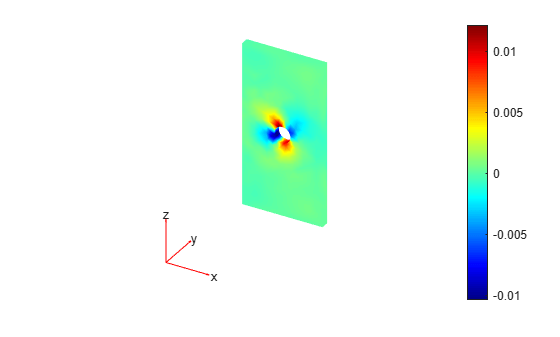`pdeplot3D(emagmodel,"ColormapData",Rmagnetostatic.MagneticPotential.Az)`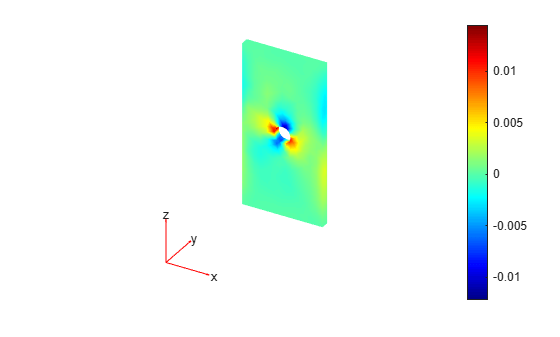Solve a magnetostatic model of a copper square with a permanent neodymium magnet in its center.

Create the unit square geometry with a circle in its center.

```L = 0.8; r = 0.25; sq = [3 4 -L L L -L -L -L L L]'; circ = [1 0 0 r 0 0 0 0 0 0]'; gd = [sq,circ]; sf = "sq + circ"; ns = char('sq','circ'); ns = ns'; g = decsg(gd,sf,ns);```

Plot the geometry with the face and edge labels.

`pdegplot(g,"FaceLabels","on","EdgeLabels","on")`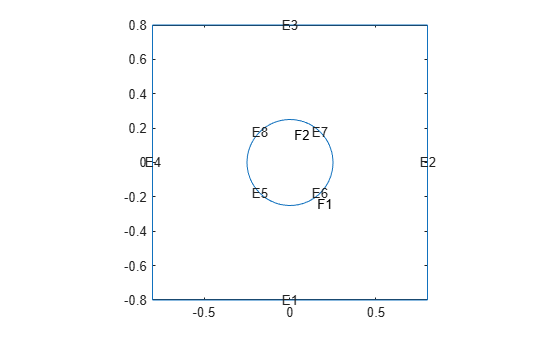Create a magnetostatic model and include the geometry in the model.

```emagmodel = createpde("electromagnetic","magnetostatic"); geometryFromEdges(emagmodel,g);```

Specify the vacuum permeability value in the SI system of units.

`emagmodel.VacuumPermeability = 1.2566370614e-6;`

Specify the relative permeability of the copper for the square.

```electromagneticProperties(emagmodel,"Face",1, ... "RelativePermeability",1);```

Specify the relative permeability of the neodymium for the circle.

```electromagneticProperties(emagmodel,"Face",2, ... "RelativePermeability",1.05);```

Specify the magnetization magnitude for the neodymium magnet.

`M = 1e6;`

Specify magnetization on the circular face in the positive x-direction. Magnetization for a 2-D model is a column vector of two elements.

```dir = [1;0]; electromagneticSource(emagmodel,"Face",2,"Magnetization",M*dir);```

Apply the magnetic potential boundary conditions on the edges framing the square.

`electromagneticBC(emagmodel,"Edge",1:4,"MagneticPotential",0);`

Generate the mesh with finer meshing near the edges of the circle.

```generateMesh(emagmodel,"Hedge",{5:8,0.007}); figure pdemesh(emagmodel)```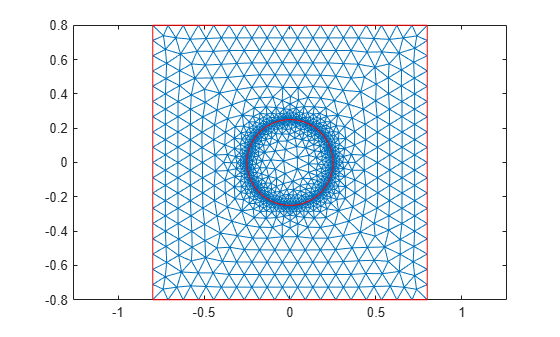Solve the model, and find the resulting magnetic fields B and H. Here, $\mathit{B}=\mu \mathit{H}+{\mu }_{0}\mathit{M}$, where $\mu$ is the absolute magnetic permeability of the material, ${\mu }_{0}$ is the vacuum permeability, and $\mathit{M}$ is the magnetization.

```R = solve(emagmodel); Bmag = sqrt(R.MagneticFluxDensity.Bx.^2 + R.MagneticFluxDensity.By.^2); Hmag = sqrt(R.MagneticField.Hx.^2 + R.MagneticField.Hy.^2);```

Plot the magnetic field B.

```figure pdeplot(emagmodel,"XYData",Bmag, ... "FlowData",[R.MagneticFluxDensity.Bx ... R.MagneticFluxDensity.By])```Plot the magnetic field H.

```figure pdeplot(emagmodel,"XYData",Hmag, ... "FlowData",[R.MagneticField.Hx R.MagneticField.Hy])```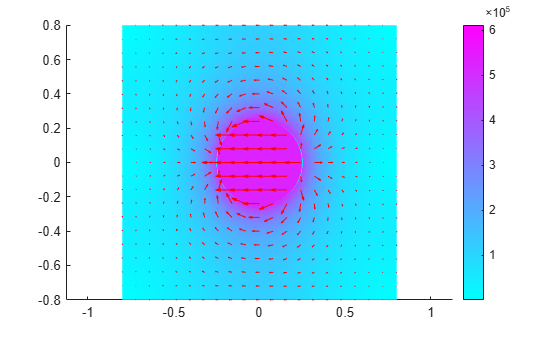## Version History

Introduced in R2021a